# Intermediate Geometry : How to find the angle for a percentage of a circle

## Example Questions

2 Next →

### Example Question #11 : Sectors

If you have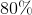of a circle, what is the angle, in degrees, that creates that region?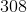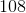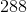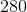Explanation:

A full circle has 360 degrees, which means that 100% of the circle is 360 degrees.

First we need to convert the percentage into a decimal.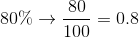If you multiply 360 by 0.80, you get the degree measure that corresponds to the percentage, which is 288.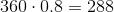### Example Question #11 : How To Find The Angle For A Percentage Of A Circle

If you have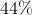of a circle, what is the angle, in degrees, that creates that region?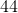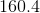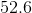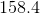Explanation:

A full circle has 360 degrees, which means that 100% of the circle is 360 degrees.

First convert the percentage into a decimal.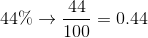If you multiply 360 by 0.44, you get the degree measure that corresponds to the percentage, which is 158.4.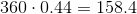### Example Question #13 : Plane Geometry

If you have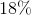of a circle, what is the angle, in degrees, that creates that region?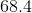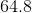Explanation:

A full circle has 360 degrees, which means that 100% of the circle is 360 degrees.

First convert the percentage into a decimal.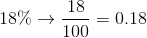If you multiply 360 by 0.18, you get the degree measure that corresponds to the percentage, which is 64.8.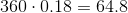### Example Question #11 : How To Find The Angle For A Percentage Of A Circle

If you have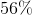of a circle, what is the angle, in degrees, that creates that region?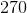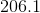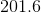Explanation:

A full circle has 360 degrees, which means that 100% of the circle is 360 degrees.

We first need to convert the percentage into a decimal.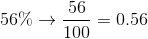If you multiply 360 by 0.56, you get the degree measure that corresponds to the percentage, which is 201.6.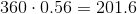2 Next →

### All Intermediate Geometry Resources